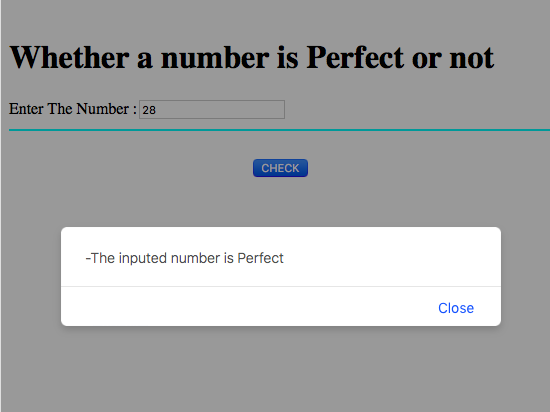# JavaScript code to check number is Perfect or not

In this JavaScript code, we are going to check whether a given number is perfect number or not.
Submitted by Aleesha Ali, on March 28, 2018

## Perfect number

A number is said to be perfect when its value is equal to its sum of complete divisors.

Example of perfect numbers are: 6, 28, 496 etc

Example:

```    Input:
Number: 6

Output:
It is a perfect number
Explanation:

Divisors of 6 are: 1, 2, 3
Sum of the divisors is: 1+2+3 =6
```

### JavaScript code to check whether a Number is Perfect or Not

```<script>
function perfectNumber()
{
number = Number(document.getElementById("N").value);
flag = number;
for(i = 0; i < number; i++)
{
remainder = number%i;
if(remainder==0)
{
}
}
{
}
else
{
window.alert("-The inputed number is not Perfect");
}
}
</script>
```

Calling JavaScript function in HTML (HTML, JS Code)

```<html>
<script>
function perfectNumber()
{
number = Number(document.getElementById("N").value);
flag = number;
for(i = 0; i < number; i++)
{
remainder = number%i;
if(remainder==0)
{
}
}
{
}
else
{
window.alert("-The inputed number is not Perfect");
}
}
</script>
<body>
<br>
<h1>Whether a number is Perfect or not</h1>
Enter The Number :<input type="text" name="n" id = "N"/>
<hr color="cyan">
<br>
<center><button onClick="perfectNumber()">CHECK</button>
</body>
</html>
```

Output